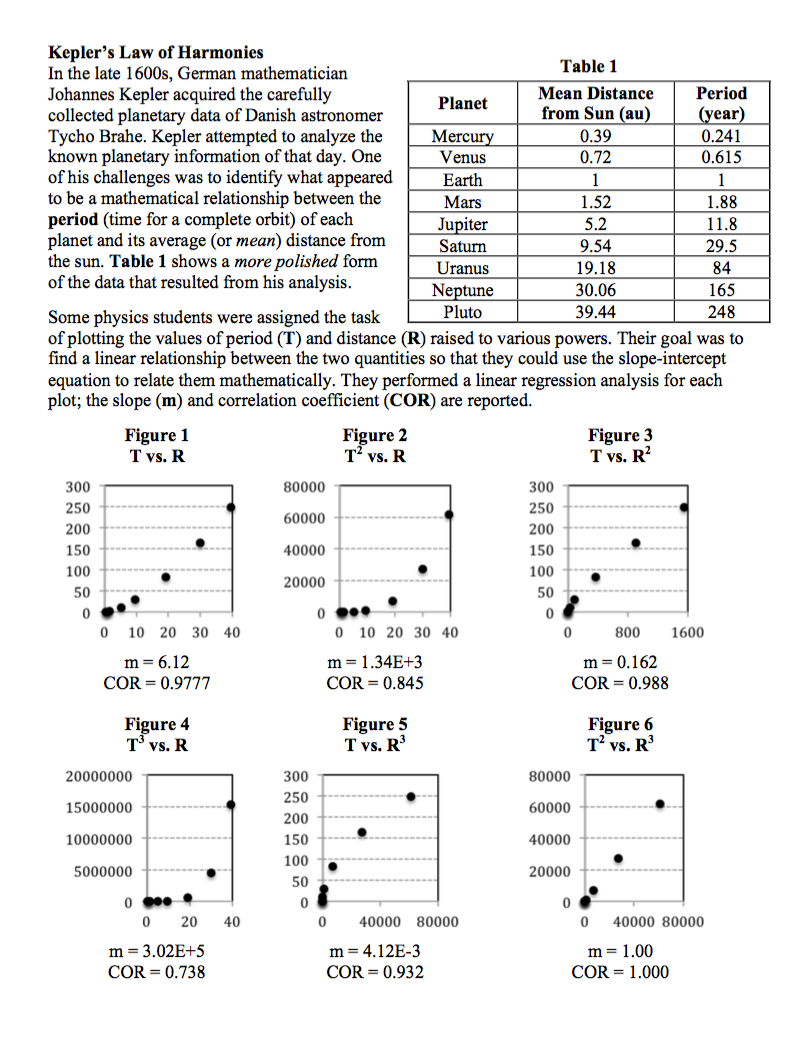###Kepler's Law of Harmonies

Resource:
The Science Reasoning Center: Kepler's Law of Harmonies

Description:
This information describes an activity in which students analyze period-radius data for the orbit of the (traditional) nine planets. The information is presented in the form of a data table and six different graphs of period and radius raised to various powers. Questions target a student's ability to understand the linear regression process, to recognize patterns in the data and graphs, to identify the relationship between the two variables, and to use the relationship to make predictions about the radius ratio or period ratio for planets in a fictional solar system.

Performance Expectation:
HS-ESS1-4:  Use mathematical or computational representations to predict the motion of orbiting objects in the solar system.

This activity aligns with the three dimensions of the Next Generation Science Standards in the manner described below:

Disciplinary Core Ideas
 Earth Science – Earth and the Solar System (HS-ESS1.B.1): Kepler's laws describe common features of the motions of orbiting objects, including elliptical paths around the sun. Orbits may change due to the gravitational effects from, or collisions with, other objects in the solar system. By analyzing six period vs. radius graphs of the planets that orbit our sun, this passages points students to ‘discover’ Kepler’s 3rd Law.  The data set helps students understand that all objects orbiting our sun obey this law of motion—a law that has great power in predicting everything from when comets will return to the distance to remote objects that orbit our sun.

Crosscutting Concepts
 Patterns: Different patterns may be observed at each of the scales at which a system is studied and can provide evidence for causality in explanations of phenomena. Like Johannes Kepler who carefully analyzed the planetary data collected by predecessor Tycho Brahe, in this passage students are asked to analyze graphs of planetary data in order to discover patterns between a planet’s orbital radius and its period of revolution around the sun.  Such connections beg an explanation as to why these patterns exists.  And while not explicitly stated in this passage, students are drawn to see the universality of the sun’s gravitational effects on all objects. Scale, Proportion, and Quantity: Algebraic thinking is used to examine scientific data and predict the effect of a change in one variable on another (e.g., linear growth vs. exponential growth). After analyzing planetary orbit data, questions at the end of this passage ask students to use algebraic thinking to predict the effects of changing a planet’s orbital radius on its period of revolution around the sun.  As students do so, they come to understand that this pattern does not just apply to planets but to any object orbiting our sun.

Practices
 Analyzing and Interpreting Data: Apply concepts of statistics and probability (including determining function fits to data, slope, intercept, and correlation coefficient for linear fits) to scientific and engineering questions and problems, using digital tools when feasible. Using graphs to linearize one quantity as a function of another quantity is an important skill in generating mathematical relationship from empirical data.  This passage provides students the opportunity to consider the correlation coefficient for linear fits in order to determine which graph best linearizes the data.  As they do, students will not only practice this important skill but they will also discover Kepler’s 3rd Law in the process! Using Mathematical and Computational Thinking: Use mathematical representations of phenomena or design solutions to describe and/or support claims and/or explanations. In addition to linearizing planetary orbital data, several questions at the close of this passage ask students to find and use proportions and then to develop a mathematical model for this data.  What is more, as students consider the end-of-passage questions, they are using the same skills that Kepler did as he discovered his “Law of Harmonies” known as Kepler’s 3rd Law.

Associated Reading from The Physics Classroom

Other Supporting Pages at The Physics Classroom:

View Infographic.
(Coming Soon)

### Search the NGSS Corner

Maybe you're looking for something really specific that pertains to a desired topic and emphasizes one or more of the listed NGSS dimensions. Why not try a search of this section of our website? Simply select from one or more of the pull-down menus and click Search  This page will reload and a collection  of possibilities will be displayed in this section of the page and sorted by relevancy.